If there is one prayer that you should pray/sing every day and every hour, it is the LORD's prayer (Our FATHER in Heaven prayer)
It is the most powerful prayer. A pure heart, a clean mind, and a clear conscience is necessary for it.
- Samuel Dominic Chukwuemeka

For in GOD we live, and move, and have our being. - Acts 17:28

The Joy of a Teacher is the Success of his Students. - Samuel Dominic Chukwuemeka

# Solved Examples on MensurationUse the Mensuration Formulas
For ACT Students
The ACT is a timed exam...$60$ questions for $60$ minutes
This implies that you have to solve each question in one minute.
Some questions will typically take less than a minute a solve.
Some questions will typically take more than a minute to solve.
The goal is to maximize your time. You use the time saved on those questions you solved in less than a minute, to solve the questions that will take more than a minute.
So, you should try to solve each question correctly and timely.
So, it is not just solving a question correctly, but solving it correctly on time.
Please ensure you attempt all ACT questions.
There is no negative penalty for any wrong answer.

For JAMB and CMAT Students
Calculators are not allowed. So, the questions are solved in a way that does not require a calculator.

For NSC Students
For the Questions:
Any space included in a number indicates a comma used to separate digits...separating multiples of three digits from behind.
Any comma included in a number indicates a decimal point.
For the Solutions:
Decimals are used appropriately rather than commas
Commas are used to separate digits appropriately. Solve all questions
Show all work

(1.) ACT A certain circle has an area of $\pi$ square inches.
How many inches long is its radius?

$A.\:\: \dfrac{1}{2} \\[5ex] B.\:\: 1 \\[3ex] C.\:\: 2 \\[3ex] D.\:\: \dfrac{\pi}{2} \\[5ex] E.\:\: \pi \\[3ex]$

$A = \pi \\[3ex] r = ? \\[3ex] r = \dfrac{\sqrt{A\pi}}{\pi} \\[5ex] = \dfrac{\sqrt{\pi * \pi}}{\pi} \\[5ex] = \dfrac{\sqrt{\pi^2}}{\pi} \\[5ex] = \dfrac{\pi}{\pi} \\[5ex] = 1\:\:inch$
(2.) JAMB A rectangular lawn has an area of $1815$ square yards.
If its length is $50$ meters, find its width in meters, given that $1$ meter equals $1.1$ yards.

$A.\:\: 39.93 \\[3ex] B.\:\: 35.00 \\[3ex] C.\:\: 33.00 \\[3ex] D.\:\: 30.00 \\[3ex]$

$A = 1815\:yd^2 \\[3ex] l = 50\:m \\[3ex] w =...to\:\:be\:\:in\:\:m \\[3ex] Convert\:\:1815\:\:yd^2\:\:to\:\:m^2 \\[3ex] 1815\:\:yd * yd * \dfrac{1\:m * 1\:m}{1.1\:yd * 1.1\:yd} \\[5ex] A = \dfrac{1815m^2}{1.21} \\[5ex] A = 1500\:m^2 \\[3ex] w = \dfrac{A}{l} \\[5ex] w = \dfrac{1500\:m^2}{50\:m} \\[5ex] w = 30\:m$
(3.) CSEC The diagram below, not drawn to scale, shows the plan of a swimming pool in the shape of a rectangle and two semicircles.
The rectangle has dimensions $8$ metres by $3.5$ metres.
$\left[Use\:\: \pi = \dfrac{22}{7}\right]\\[3ex]$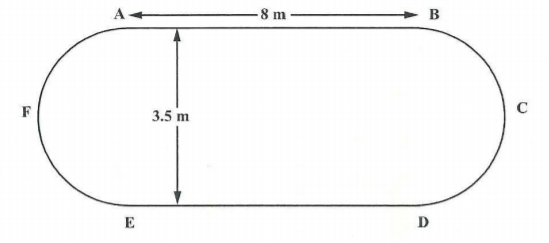(i) State the length of the diameter of the semicircle, $AFE$
(ii) Calculate the perimeter of the swimming pool.

The diameter of the semicircle is the width of the rectangle

$(i) \\[3ex] diameter\:\:of\:\:semicircle = 3.5\:m \\[3ex]$ The perimeter of the swimming pool is the sum of:
Circumference of the semicircle $AFE$ and
Length of the rectangle $ED$ and
Circumference of the semicircle $DCB$ and
Length of the rectangle $BA$

$(ii) \\[3ex] Circumference\:\:of\:\:semicircle\:\:AFE = \dfrac{\pi d}{2} \\[5ex] Circumference\:\:of\:\:semicircle\:\:DCB = \dfrac{\pi d}{2} \\[5ex] Length\:\:of\:\:rectangle\:\:ED = 8\:m \\[3ex] Length\:\:of\:\:rectangle\:\:BA = 8\:m \\[3ex] Perimeter\:\:of\:\:the\:\:swimming\:\:pool \\[3ex] = \dfrac{\pi d}{2} + \dfrac{\pi d}{2} + 8 + 8 \\[5ex] = \pi d + 16 \\[3ex] = \dfrac{22}{7} * 3.5 + 16 \\[5ex] = \dfrac{22}{7} * \dfrac{7}{2} + 16 \\[5ex] = 11 + 16 \\[3ex] = 27\:m$
(4.) ACT The length of a rectangle is $(x + 5)$ units and its width is $(x + 7)$ units.
Which of the following expresses the remaining area of the rectangle, in square units, if a square, $x$ units in length, is removed from the interior of the rectangle?

$F.\:\: 12 \\[3ex] G.\:\: 35 \\[3ex] H.\:\: 2x + 12 \\[3ex] J.\:\: 12x + 35 \\[3ex] K.\:\: x^2 + 12x + 35 \\[3ex]$

$Area\:\:of\:\:rectangle \\[3ex] = (x + 5)(x + 7) \\[3ex] = x^2 + 7x + 5x + 35 \\[3ex] = x^2 + 12x + 35 \\[3ex] Area\:\:of\:\:square \\[3ex] = x^2 \\[3ex] Remaining\:\:area\:\:of\:\:rectangle \\[3ex] = x^2 + 12x + 35 - x^2 \\[3ex] = 12x + 35$
(5.) ACT What is the area, in square meters, of a right triangle with sides of length $8$ meters, $15$ meters and $17$ meters?

$F.\:\: 40 \\[3ex] G.\:\: 60 \\[3ex] H.\:\: 68 \\[3ex] J.\:\: 120 \\[3ex] K.\:\: 127\dfrac{1}{2} \\[5ex]$

Because it is a right triangle, the hypotenuse is $17$ meters (longest side)
This implies that the other two sides are the base and the height.
Any of those two sides could be either the base or the height. No information in the question suggets otherwise.

$\underline{Short\:\:Method:\:\:Recommended\:\:for\:\:the\:\:ACT} \\[3ex] A = \dfrac{1}{2} * b * h \\[5ex] A = \dfrac{1}{2} * 8 * 15 \\[5ex] A = 4 * 15 \\[3ex] A = 60\:m^2 \\[3ex] \underline{Long\:\:Method:\:\:Hero's\:\:Formula} \\[3ex] Semiperimeter = \dfrac{height + base + hypotenuse}{2} \\[5ex] Semiperimeter = \dfrac{15 + 8 + 17}{2} = \dfrac{40}{2} = 20\:cm \\[5ex] first\:\:difference = Semiperimeter - height = 20 - 15 = 5\:cm \\[3ex] second\:\:difference = Semiperimeter - base = 20 - 8 = 12\:cm \\[3ex] third\:\:difference = Semiperimeter - hypotenuse = 20 - 17 = 3\:cm \\[3ex] Area = \sqrt{Semiperimeter * first\:\:difference * second\:\:difference * third\:\:difference} \\[3ex] Area = \sqrt{20 * 5 * 12 * 3} \\[3ex] Area = \sqrt{3600\:m^4} \\[3ex] Area = 60\:m^2$
(6.) ACT The $2$ squares in the figure below have the same dimensions.
The vertex of one square is at the center of the other square.
What is the area of the shaded region, in square inches?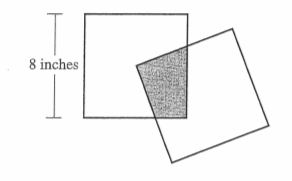$A.\:\: 4 \\[3ex] B.\:\: 8 \\[3ex] C.\:\: 16 \\[3ex] D.\:\: 32 \\[3ex] E.\:\: 64 \\[3ex]$

The vertex of one square is at the center of the other square.
Do not let the titled square confuse you.
If you stand it directly, it has the same area as when it is titled because it's vertex is at the center of the other square.
This implies that half of the length of the titled square is at the center of the other square.
The $2$ squares in the figure below have the same dimensions.
This implies that $\dfrac{1}{2} * 8 = 4\:inches$ of the titled square is at the center of the other square.

$Area\:\:of\:\:the\:\:shaded\:\:region \\[3ex] = 4(4) \\[3ex] = 16\:in^2 \\[3ex]$ Alternatively, one can say that the area of the shaded region (region of the titled square) is one-quarter of the area of the first square.

$Area\:\:of\:\:the\:\:first\:\:square = 8(8) = 64\:in^2 \\[3ex] Area\:\:of\:\:the\:\:shaded\:\:region = \dfrac{1}{4} * 64 \\[3ex] = 16\:in^2$
(7.) WASSCE The curved surface areas of two cones are equal.
The base radius of one is $5\:cm$ and its slant height is $12\:cm$
Calculate the height of the second cone if its base radius is $6\:cm$

$\underline{First\:\:Cone} \\[3ex] r = 5\:cm \\[3ex] l = 12\:cm \\[3ex] LSA = \pi rl = \pi (5)(12) = 60\pi cm^2 \\[3ex] \underline{Second\:\:Cone} \\[3ex] r = 6\:cm \\[3ex] LSA = 60\pi cm^2...from\:\:the\:\:question:\:\:curved\:\:surface\:\:areas\:\:are\:\:equal \\[3ex] h = ? \\[3ex] h = \dfrac{\sqrt{LSA^2 - \pi^2 r^4}}{\pi r} \\[5ex] = \dfrac{\sqrt{(60\pi)^2 - \pi^2 * 6^4}}{\pi * 6} \\[5ex] = \dfrac{\sqrt{3600\pi^2 - 1296\pi^2}}{6\pi} \\[5ex] = \dfrac{\sqrt{2304\pi^2}}{6\pi} \\[5ex] = \dfrac{48\pi}{6\pi} \\[5ex] = 8 \\[3ex] h = 8\:cm$
(8.)

The vertex of one square is at the center of the other square.
Do not let the titled square confuse you.
If you stand it directly, it has the same area as when it is titled because it's vertex is at the center of the other square.
This implies that half of the length of the titled square is at the center of the other square.
The $2$ squares in the figure below have the same dimensions.
This implies that $\dfrac{1}{2} * 8 = 4\:inches$ of the titled square is at the center of the other square.

$Area\:\:of\:\:the\:\:shaded\:\:region \\[3ex] = 4(4) \\[3ex] = 16\:in^2 \\[3ex]$ Alternatively, one can say that the area of the shaded region (region of the titled square) is one-quarter of the area of the first square.

$Area\:\:of\:\:the\:\:first\:\:square = 8(8) = 64\:in^2 \\[3ex] Area\:\:of\:\:the\:\:shaded\:\:region = \dfrac{1}{4} * 64 \\[3ex] = 16\:in^2$
(9.) WASSCE A container, in the form of a cone resting on its vertex, is full when $4.158$ litres of water is poured into it.
(a) If the radius of its base is $21\:cm$,
(i) represent the information in a diagram;
(ii) calculate the height of the container.

(b) A certain amount of water is drawn out of the container such that the surface diameter of the water drops to $28\:cm$.
Calculate the volume of the water drawn out. $\left(Take\:\:\pi = \dfrac{22}{7}\right)$

(a)
(i)
The diagram can be represented as: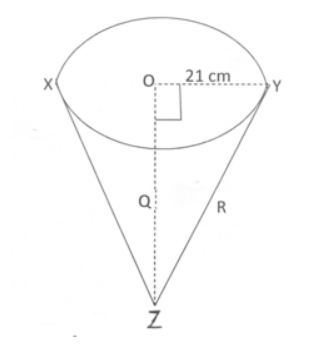$(ii) \\[3ex] r = 21\:cm \\[3ex] V = 4.158\:litres = 4.158\:dm^3 \\[3ex] Convert\:\:to\:\:cm^3 \\[3ex] 4.158\:dm^3 \\[3ex] = 4.158\:dm * dm * dm * \dfrac{0.1\:m * 0.1\:m * 0.1\:m}{1\:dm * 1\:dm * 1\:dm} * \dfrac{1\:cm * 1\:cm * 1\:cm}{0.01\:m * 0.01\:m * 0.01\:m} \\[5ex] = \dfrac{4.158 * 0.1 * 0.1 * 0.1 * cm^3}{0.01 * 0.01 * 0.01} \\[5ex] = \dfrac{0.001458\:cm^3}{0.000001} \\[5ex] = 4158\:cm^3 \\[3ex] h = \dfrac{3V}{\pi r^2} \\[5ex] = 3V \div \pi r^2 \\[3ex] = 3(4158) \div \left(\dfrac{22}{7} * 21 * 21\right) \\[5ex] = 3(4158) \div (22 * 3 * 21) \\[3ex] = \dfrac{3 * 4158}{22 * 3 * 21} \\[5ex] = \dfrac{4158}{462} \\[5ex] = 9\:cm \\[3ex] (b) \\[3ex] Volume\:\:of\:\:cone = 4158\:cm^3 \\[3ex] Water\:\:was\:\:drawn\:\:out \\[3ex] \underline{Remaining\:\:volume\:\:of\:\:water} \\[3ex] d_{remain} = 28\:cm \\[3ex] r_{remain} = \dfrac{d_{remain}}{2} \\[5ex] r_{remain} = \dfrac{28}{2} = 14\:cm \\[5ex] \dfrac{h_{remain}}{h} = \dfrac{r_{remain}}{r}...Similar\:\:\triangle s \\[5ex] \dfrac{h_{remain}}{9} = \dfrac{14}{21} \\[5ex] h_{remain} = \dfrac{9 * 14}{21} = 6\:cm \\[5ex] V_{remain} = \dfrac{\pi * r_{remain}^2 * h_{remain}}{3} \\[5ex] V_{remain} = \dfrac{1}{3} * \dfrac{22}{7} * 14 * 14 * 6 \\[5ex] V_{remain}= 22 * 2 * 14 * 2 \\[3ex] V_{remain} = 1232\:cm^3 \\[3ex] \underline{Volume\:\:of\:\:water\:\:drawn\:\:out} \\[3ex] V_{drawn} + V_{remain} = V \\[3ex] V_{drawn} = V - V_{remain} \\[3ex] V_{drawn} = 4158 - 1232 \\[3ex] V_{drawn} = 2926\:cm^3$
(10.) ACT A floor has the dimensions shown below.
How many square feet of carpeting are needed to cover the entire floor?
(Note: All angles are right angles.)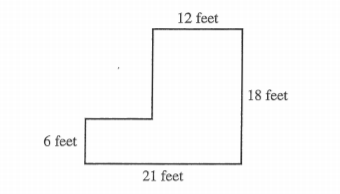$A.\:\: 57 \\[3ex] B.\:\: 72 \\[3ex] C.\:\: 162 \\[3ex] D.\:\: 270 \\[3ex] E.\:\: 324 \\[3ex]$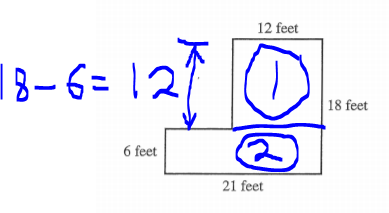The square feet of carpeting needed to cover the entire floor is the area of the composite shape.
The area of the composite shape is the sum of the area of section $1$ and the area of section $2$

$Area\:\:of\:\:section\:1 = 12(12) = 144\:ft^2 \\[3ex] Area\:\:of\:\:section\:2 = 6(21) = 126\:ft^2 \\[3ex] Area\:\:of\:\:composite\:\:shape = 144 + 126 = 270\:ft^2 \\[3ex]$ $270$ square feet of carpeting are needed to cover the entire floor.
(11.)

(12.) ACT How long, in centimeters, is $1$ side of a square whose perimeter is equal to the circumference of a circle with a radius of $2$ centimeters?

$F.\;\; \pi \\[3ex] G.\;\; \dfrac{\pi}{2} \\[5ex] H.\;\; 4\pi \\[3ex] J.\;\; 16\pi \\[3ex] K.\;\; 4 \\[3ex]$

$\underline{Square} \\[3ex] Let\;\;side = x \\[3ex] Perimeter = 4 * side \\[3ex] P = 4x \\[3ex] \underline{Circle} \\[3ex] radius = 2\;cm \\[3ex] Circumference = 2 * \pi * radius \\[3ex] C = 2 * \pi * 2 \\[3ex] C = 4\pi \\[3ex] \underline{Question} \\[3ex] P = C \\[3ex] 4x = 4\pi \\[3ex] x = \dfrac{4\pi}{4} \\[5ex] x = \pi \\[3ex]$ The length of a side of the square is $\pi$ centimeters
(13.)

(14.) ACT One side of square $ABCD$ has a length of $15$ meters.
A certain rectangle whose area is equal to the area of $ABCD$ has a width of $10$ meters.
What is the length, in meters, of the rectangle?

$F.\;\; 15 \\[3ex] G.\;\; 20 \\[3ex] H.\;\; 22.5 \\[3ex] J.\;\; 25 \\[3ex] K.\;\; 37.5 \\[3ex]$

$\underline{Square\;ABCD} \\[3ex] Side = 15 \\[3ex] Area = Side^2 \\[3ex] A_{square} = 15^2 = 225 \\[3ex] \underline{Rectangle} \\[3ex] width = 10\;meters \\[3ex] length = p \\[3ex] Area = length * width \\[3ex] A_{rectangle} = p * 10 = 10p \\[3ex] \underline{Question} \\[3ex] A_{rectangle} = A_{square} \\[3ex] 10p = 225 \\[3ex] p = \dfrac{225}{10} \\[5ex] p = 22.5 \\[3ex]$ The length of the rectangle is $22.5$ meters
(15.)

(16.) ACT A rectangular stage is $90$ feet long and $30$ feet wide.
What is the area, in square yards, of this stage?

$A.\;\; 30\sqrt{3} \\[3ex] B.\;\; 300 \\[3ex] C.\;\; 675 \\[3ex] D.\;\; 900 \\[3ex] E.\;\; 1,700 \\[3ex]$

$Area\:\;in\:\:square\:\:feet = 90\:feet * 30\:feet = 2700\:square\:feet \\[3ex] Convert\:\: 2700\:feet^2 \:\:to\:\: yard^2 \\[3ex] \underline{First\:\:Method:\:\:Unity\:\:Fraction\:\:Method} \\[3ex] 2700\:ft^2\:\:to\:\:yd^2 \\[3ex] 2700\:ft * ft * \dfrac{.....yd}{.....ft} * \dfrac{.....yd}{.....ft} \\[5ex] 2700\:ft * ft * \dfrac{1\:yd}{3\:ft} * \dfrac{1\:yd}{3\:ft} \\[5ex] = \dfrac{2700 * 1 * 1}{3 * 3} \\[5ex] = \dfrac{2700}{9} \\[5ex] = 300\:yd^2 \\[3ex]$ Second Method: Proportional Reasoning Method

Let $c$ = converted unit from $ft^2$ to $yd^2$

$3\:ft = 1\:yd \\[3ex] Square\:\:both\:\:sides \\[3ex] (3\:ft)^2 = (1\:yd)^2 \\[3ex] 3^2 * ft^2 = 1^2 * yd^2 \\[3ex] 9\:ft^2 = 1\:yd^2 \\[3ex]$
$ft^2$ $yd^2$
$9$ $1$
$2700$ $c$

$\dfrac{c}{1} = \dfrac{2700}{9} \\[5ex] c = 300\:yd^2 \\[3ex] \therefore 2700\:ft^2 = 300\:yd^2 \\[3ex]$ The area of the rectangular stage is $300$ square yards
(17.)

(18.) ACT What is the ratio of the diameter to the circumference of a circle?

$F.\:\: \dfrac{1}{2\pi} \\[5ex] G.\:\: \dfrac{1}{\pi} \\[5ex] H.\:\: \dfrac{\pi}{2} \\[5ex] J.\:\: \pi \\[3ex] K.\:\: 2\pi \\[3ex]$

$d = 2r \\[3ex] C = 2\pi r \\[3ex] \dfrac{d}{C} = \dfrac{2r}{2\pi r} \\[5ex] \dfrac{d}{C} = \dfrac{1}{\pi}$
(19.)

(20.)

(21.)

(22.) ACT The track for a model railroad display is set up as 2 circles that are tangent to one another and have diameters of 30 feet and 50 feet, respectively, as shown below.
The engine of the train travels at a constant rate of 75 feet per minute.
To the nearest minute, how many minutes does the engine take to go in a figure 8 pattern around the entire track exactly 1 time?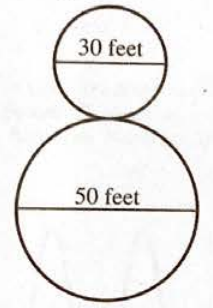$F.\;\; 1 \\[3ex] G.\;\; 2 \\[3ex] H.\;\; 3 \\[3ex] J.\;\; 4 \\[3ex] K.\;\; 7 \\[3ex]$

Figure 8 pattern around the entire track exactly 1 time is the circumference of the 2 circles

$C = \pi d \\[3ex] \underline{Small\;\;circle} \\[3ex] d = 30\;feet \\[3ex] C = 30\pi \\[3ex] \underline{Large\;\;circle} \\[3ex] d = 50\;feet \\[3ex] C = 50\pi \\[3ex] \underline{Figure\;\;8\;\;pattern} \\[3ex] C = 30\pi + 50\pi = 80\pi \\[3ex]$
Proportional Reasooning Method to calculate speed
Distance (feet) Time (minute)
$75$ $1$
$80\pi$ $p$

$\dfrac{x}{1} = \dfrac{80\pi}{75} \\[5ex] x = 80 * \dfrac{22}{7} * \dfrac{1}{75} \\[5ex] x = \dfrac{1760}{525} \\[5ex] x = 3.352380952\; feet \\[3ex] x \approx 3\;minutes$
(23.)

(24.)